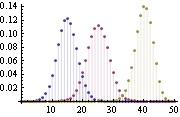# equivalent of PlotFilling->Axis

Is there a way to create a line plot similar to Mathematica’s PlotFilling->Axis option for ListPlot?

It is a plot of a vector as a line plot, but for each point, there is a vertical line dropped down to the axis. It is demonstrated on the following link:

http://reference.wolfram.com/mathematica/ref/Filling.html

With the example given:

ListPlot[Table[{k,
PDF[BinomialDistribution[50, p], k]}, {p, {0.3, 0.5, 0.8}}, {k, 0,
50}], Filling -> Axis]

Thanks!

Art wrote:

Is there a way to create a line plot similar to Mathematica's PlotFilling->Axis option for ListPlot?

Like this?

http://matplotlib.sourceforge.net/examples/pylab_examples/fill_between_demo.html

Eric

···

It is a plot of a vector as a line plot, but for each point, there is a vertical line dropped down to the axis. It is demonstrated on the following link:

http://reference.wolfram.com/mathematica/ref/Filling.html

With the example given:

ListPlot[Table[{k,
PDF[BinomialDistribution[50, p], k]}, {p, {0.3, 0.5, 0.8}}, {k, 0,
50}], Filling -> Axis]

Thanks!

------------------------------------------------------------------------

------------------------------------------------------------------------------

------------------------------------------------------------------------

_______________________________________________
Matplotlib-users mailing list
Matplotlib-users@lists.sourceforge.net
https://lists.sourceforge.net/lists/listinfo/matplotlib-users

Hi Eric,

I was thinking more like the tiny attachment (hope attachments are ok). Basically, given a list of (x,y) coordinates, plot the (x,y) point as a little circle and drop a line down from the point to the x-axis (but not fill the whole region under the plot).

It’s very nice for plotting a sparse signal, where most of the y’s are equal to zero but some are not.

Thanks,
Art.···

On Wed, Jun 24, 2009 at 7:18 PM, Eric Firing <efiring@…202…> wrote:

Art wrote:

Is there a way to create a line plot similar to Mathematica’s PlotFilling->Axis option for ListPlot?

Like this?

http://matplotlib.sourceforge.net/examples/pylab_examples/fill_between_demo.html

Eric

It is a plot of a vector as a line plot, but for each point, there is a vertical line dropped down to the axis. It is demonstrated on the following link:

http://reference.wolfram.com/mathematica/ref/Filling.html

With the example given:

ListPlot[Table[{k,

PDF[BinomialDistribution[50, p], k]}, {p, {0.3, 0.5, 0.8}}, {k, 0,

50}], Filling -> Axis]

Thanks!

Matplotlib-users mailing list

Matplotlib-users@lists.sourceforge.net

https://lists.sourceforge.net/lists/listinfo/matplotlib-users

I guess the stem plot is close to what you need.

http://matplotlib.sourceforge.net/examples/pylab_examples/stem_plot.html

Regards,

-JJ

···

On Wed, Jun 24, 2009 at 3:32 PM, Art<grenander@...287...> wrote:

Hi Eric,

I was thinking more like the tiny attachment (hope attachments are ok).
Basically, given a list of (x,y) coordinates, plot the (x,y) point as a
little circle and drop a line down from the point to the x-axis (but not
fill the whole region under the plot).

It's very nice for plotting a sparse signal, where most of the y's are equal
to zero but some are not.

Thanks,
Art.

On Wed, Jun 24, 2009 at 7:18 PM, Eric Firing <efiring@...202...> wrote:

Art wrote:

Is there a way to create a line plot similar to Mathematica's
PlotFilling->Axis option for ListPlot?

Like this?

http://matplotlib.sourceforge.net/examples/pylab_examples/fill_between_demo.html

Eric

It is a plot of a vector as a line plot, but for each point, there is a
vertical line dropped down to the axis. It is demonstrated on the following

http://reference.wolfram.com/mathematica/ref/Filling.html

With the example given:

ListPlot[Table[{k,
PDF[BinomialDistribution[50, p], k]}, {p, {0.3, 0.5, 0.8}}, {k, 0,
50}], Filling -> Axis]

Thanks!

------------------------------------------------------------------------

------------------------------------------------------------------------------

------------------------------------------------------------------------

_______________________________________________
Matplotlib-users mailing list
Matplotlib-users@lists.sourceforge.net
https://lists.sourceforge.net/lists/listinfo/matplotlib-users

------------------------------------------------------------------------------

_______________________________________________
Matplotlib-users mailing list
Matplotlib-users@lists.sourceforge.net
https://lists.sourceforge.net/lists/listinfo/matplotlib-users

Thanks! I knew it had to exist and with a lot of nice options, as usual.

Art

···

On Wed, Jun 24, 2009 at 10:21 PM, Jae-Joon Lee <lee.j.joon@…287…> wrote:

I guess the stem plot is close to what you need.

http://matplotlib.sourceforge.net/examples/pylab_examples/stem_plot.html

Regards,

-JJ

On Wed, Jun 24, 2009 at 3:32 PM, Art<grenander@…287…> wrote:

Hi Eric,

I was thinking more like the tiny attachment (hope attachments are ok).

Basically, given a list of (x,y) coordinates, plot the (x,y) point as a

little circle and drop a line down from the point to the x-axis (but not

fill the whole region under the plot).

It’s very nice for plotting a sparse signal, where most of the y’s are equal

to zero but some are not.

Thanks,

Art.

On Wed, Jun 24, 2009 at 7:18 PM, Eric Firing <efiring@…202…> wrote:

Art wrote:

Is there a way to create a line plot similar to Mathematica’s

PlotFilling->Axis option for ListPlot?

Like this?

http://matplotlib.sourceforge.net/examples/pylab_examples/fill_between_demo.html

Eric

It is a plot of a vector as a line plot, but for each point, there is a

vertical line dropped down to the axis. It is demonstrated on the following

http://reference.wolfram.com/mathematica/ref/Filling.html

With the example given:

ListPlot[Table[{k,

PDF[BinomialDistribution[50, p], k]}, {p, {0.3, 0.5, 0.8}}, {k, 0,

50}], Filling -> Axis]

Thanks!

Matplotlib-users mailing list

Matplotlib-users@lists.sourceforge.net

https://lists.sourceforge.net/lists/listinfo/matplotlib-users

Matplotlib-users mailing list

Matplotlib-users@lists.sourceforge.net

https://lists.sourceforge.net/lists/listinfo/matplotlib-users